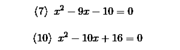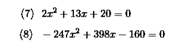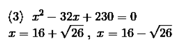# Home | Mathematics | Pre-Algebra

Wikipedia, the free encyclopedia

In algebra, a quadratic equation (from the Latin quadratus for "square") is any equation that can be rearranged in standard form as

ax2 + bx + c = 0
where x represents an unknown, and a, b, and c represent known numbers, where a &ne; 0. If a = 0, then the equation is linear, not quadratic, as there is no ax2 term. The numbers a, b, and c are the coefficients of the equation and may be distinguished by calling them, respectively, the quadratic coefficient, the linear coefficient and the constant or free term.

The values of x that satisfy the equation are called solutions of the equation, and roots or zeros of its left-hand side. A quadratic equation has at most two solutions. If there is no real solution, there are two complex solutions. If there is only one solution, one says that it is a double root. A quadratic equation always has two roots, if complex roots are included and a double root is counted for two. A quadratic equation can be factored into an equivalent equation

ax2 + bx + c = a ( x - r ) ( x - s ) = 0

where r and s are the solutions for x. Completing the square on a quadratic equation in standard form results in the quadratic formula, which expresses the solutions in terms of a, b, and c. Solutions to problems that can be expressed in terms of quadratic equations were known as early as 2000 BC.

Because the quadratic equation involves only one unknown, it is called "univariate". The quadratic equation only contains powers of x that are non-negative integers, and therefore it is a polynomial equation. In particular, it is a second-degree polynomial equation, since the greatest power is two.

A quadratic equation with real or complex coefficients has two solutions, called roots. These two solutions may or may not be distinct, and they may or may not be real.Parameters and roots:Parameter a=1Parameter a≠1Solutions with root answersComplex solutions with fraction and root answers Paper size: US Letter (8.5 x 11 in)   A4 (210 x 297 mm)